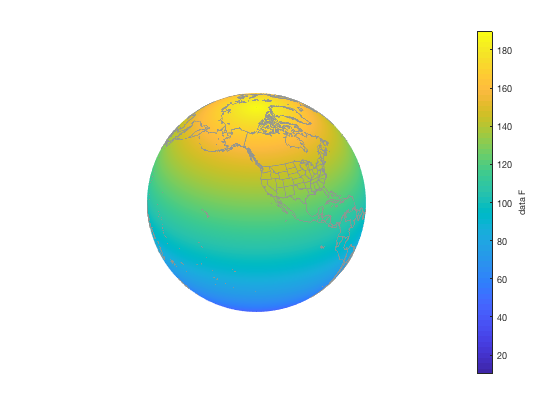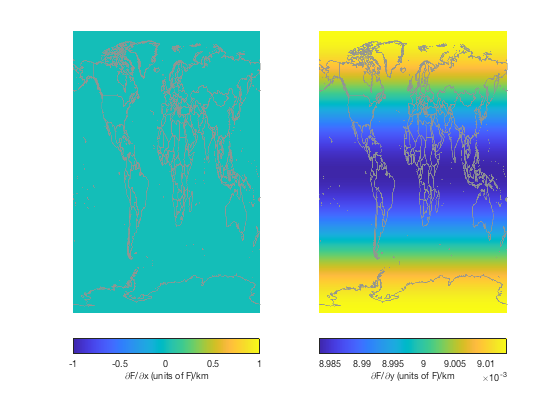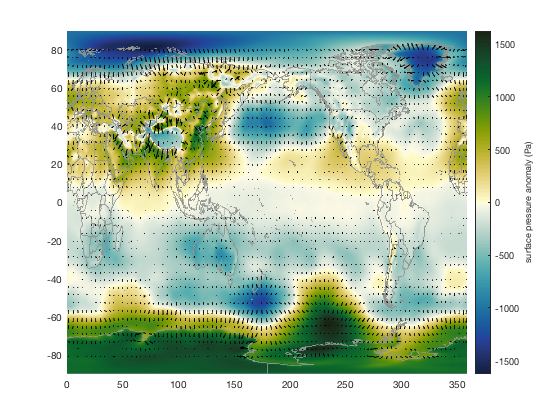cdtgradient calculates the spatial gradient of gridded data equally spaced in geographic coordinates.

## Syntax

```[FX,FY] = cdtgradient(lat,lon,F)

## Description

[FX,FY] = cdtgradient(lat,lon,F) for the gridded variable F and corresponding geographic coordinates lat and lon, cdtgradient calculates FX, the spatial rate of west-to-east change in F per meter along the Earth's surface, and FY, the south-to-north change in F per meter. This function assumes an ellipsoidal Earth as modeled by the cdtdim and earth_radius. A positive value of FX indicates that F increases from west to east in that grid cell, as a positive value of FY indicates F increases from south to north. F can be a 2D or 3D matrix whose first two dimensions must correspond to lat and lon. If F is 3D, outputs FX and FY will also be 3D, with each grid along the third dimension calculated separately.

[FX,FY] = cdtgradient(lat,lon,F,'km') returns gradients per kilometer rather than the default meters.

## Example 1: Theory

Here's a global grid of some variable F, which is only a function of latitude and increases from south to north at a rate of 1 unit F per degree of latitude. Use cdtgrid to create the grid and then define F as the value of the latitude plus 100:

```% Create a quarter-degree grid:
[lat,lon] = cdtgrid(0.25);

% Define F:
F = lat + 100;

% Plot F on a globe:
figure
globepcolor(lat,lon,F)
globeborders('color',rgb('gray'))
axis tight
cb = colorbar;
ylabel(cb,'data F')
```In the figure above we see that the range of F values go from about 10 near the South Pole to about 190 near the North Pole. That's because F is just the latitude of each grid cell plus 100.

With this dataset we know that at any given latitude, F is the same for all longitudes. That means Fx should be zero everywhere on the globe, because F never changes from west to east.

However, F does change from south to north, at a rate of 1 unit F per degree latitude. It's handy to know that degrees of latitude are separated by about 111 km (from the original definition of the meter, which said the distance from the Equator to the North Pole is 10 million meters). So if each degree of latitude is separated by 111 km, and F increases by 1 unit for each degree of latitude, then Fy should be 1/111 = 0.009 everwhere on the globe. Let's see:

```[Fx,Fy] = cdtgradient(lat,lon,F,'km');

figure
subplot(1,2,1)
pcolor(lon,lat,Fx)
borders('countries','color',rgb('gray'))
axis off % removes ticks
cb1=colorbar('location','southoutside');
xlabel(cb1,'\partialF/\partialx (units of F)/km')

subplot(1,2,2)
pcolor(lon,lat,Fy)
borders('countries','color',rgb('gray'))
axis off % removes ticks
cb2=colorbar('location','southoutside');
xlabel(cb2,'\partialF/\partialy (units of F)/km')
```Above, we see that our predictions fared pretty well. Fx is zero everywhere in the world, as we predicted, and the values of Fy center on our predicted value of 9e-3. There is some latitudinal variation in Fy, however, because the Earth is not a perfect sphere, but an ellipsoid whose degrees of latitude are not perfectly spaced by 111,111 m, as the original definition of the meter might have you believe.

## Example 2: Reality

For this example, we'll use the example surface pressure data that comes with CDT. Start by loading it:

```filename = 'ERA_Interim_2017.nc';
sp = ncread(filename,'sp'); % surface pressure
[Lat,Lon] = meshgrid(lat,lon);
```

The absolute surface pressure isn't particularly interesting because it mostly tracks surface elevation. But surface pressure anomalies are quite a bit more interesting because they give us an idea of how far out of balance the system is at a given point in time.

For convenience, let's calculate the surface pressure anomaly for January of 2017 as the surface pressure field for that month, minus the mean surface pressure from all of 2017:

```% Surface pressure "anomaly":
spa = sp(:,:,1)-mean(sp,3);
```

Here's the surface pressure anomaly we want to analyze:

```figure
globepcolor(Lat,Lon,spa);
globeborders('color',rgb('gray'))
axis tight
cmocean('delta','pivot') % sets colormap
cb = colorbar;
ylabel(cb,'surface pressure anomaly (Pa)')
view(125,5) % sets viewing angle
```Calculate the zonal and meridional surface pressure gradients in Pa/km like this

```[Sx,Sy] = cdtgradient(Lat,Lon,spa,'km');

figure
subplot(1,2,1)
pcolor(Lon,Lat,Sx)
borders('countries','color',rgb('gray'))
axis off % removes ticks
cb1=colorbar('location','southoutside');
caxis([-1 1])
cmocean diff

subplot(1,2,2)
pcolor(Lon,Lat,Sy)
borders('countries','color',rgb('gray'))
axis off % removes ticks
cb2=colorbar('location','southoutside');
caxis([-1 1])
cmocean diff
```The figure above may be somewhat difficult to interpret, so we'll take a different approach. Instead of plotting Sx and Sy as color, plot them as vectors atop the surface pressure anomaly map:

```figure
pcolor(Lon,Lat,spa)
hold on
borders('countries','color',rgb('gray'),'center',180)
cmocean('delta','pivot') % sets colormap
cb = colorbar;
ylabel(cb,'surface pressure anomaly (Pa)')

quiversc(Lon,Lat,Sx,Sy,'k','density',100)
```In the map above, we see that gradient vectors always point from low values to high values. Naturally, that's the opposite direction of the pressure gradient force.

## Author Info

This function is part of the Climate Data Toolbox for Matlab. The function and supporting documentation were written by Chad A. Greene of the University of Texas at Austin.